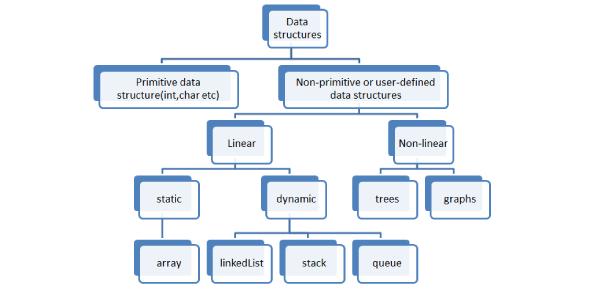# Data Structure Basic Questions Quiz! Test

10 Questions | Total Attempts: 402SettingsWhat do you know about data structure? Do you suppose you can make the grade on this quiz? Anything that can store data can be referred to as a data structure. Data structures are programmed to store ordered information so that various operations can be performed quickly. It represents the knowledge of data to be organized in memory. Take this quiz and see how much you know about data structure.

• 1.
A linear collection of data elements where the linear node is given by means of the pointer is
• A.

• B.

Node list

• C.

Primitive list

• D.

None of these

• 2.
Representation of data structure in memory is known as:
• A.

Recursive

• B.

Abstract data type

• C.

Storage structure

• D.

file structure

• 3.
An adjacency matrix representation of a graph cannot contain information of :
• A.

Nodes

• B.

Edges

• C.

Direction of edges

• D.

Parallel edges

• 4.
Quicksort is also known as
• A.

Merge sort

• B.

Heap sort

• C.

Bubble sort

• D.

None of these

• 5.
Which of the following data structure is linear data structure?
• A.

Trees

• B.

Graphs

• C.

Arrays

• D.

None of above

• 6.
The best average behaviour is shown by
• A.

Quick Sort

• B.

Merge Sort

• C.

Insertion Sort

• D.

Heap Sort

• 7.
A queue is a,
• A.

FIFO (First In First Out) list.

• B.

LIFO (Last In First Out) list.

• C.

Ordered array.

• D.

Linear tree.

• 8.
Which data structure is needed to convert infix notation to postfix notation?
• A.

Branch

• B.

Queue

• C.

Tree

• D.

Stack

• 9.
The postfix form of the expression (A+ B)*(C*D− E)*F / G is
• A.

AB+ CD*E − FG /**

• B.

AB + CD* E − F **G /

• C.

AB + CD* E − *F *G /

• D.

AB + CDE * − * F *G /

• 10.
A full binary tree with n leaves contains
• A.

N nodes.

• B.

Log n 2 nodes.

• C.

2n –1 nodes.

• D.

n 2 nodes.

Related TopicsBack to top